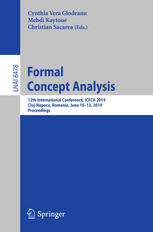• A
• A
• A
• ABC
• ABC
• ABC
• А
• А
• А
• А
• А
Regular version of the site

## Scalable Estimates of Concept Stability

P. 157-172.

Data mining aims at finding interesting patterns from datasets, where “interesting” means reflecting intrinsic dependencies in the domain of interest rather than just in the dataset. Concept stability is a popular relevancy measure in FCA. Experimental results of this paper show that high stability of a concept for a context derived from the general population suggests that concepts with the same intent in other samples drawn from the population have also high stability. A new estimate of stability is introduced and studied. It is experimentally shown that the introduced estimate gives a better approximation than the Monte Carlo approach introduced earlier.

### In bookVol. 8478. Springer, 2014.